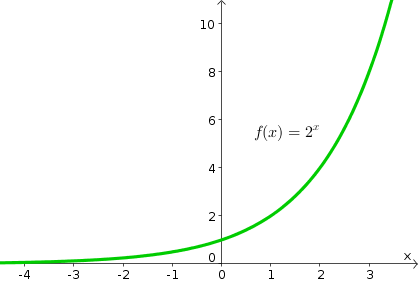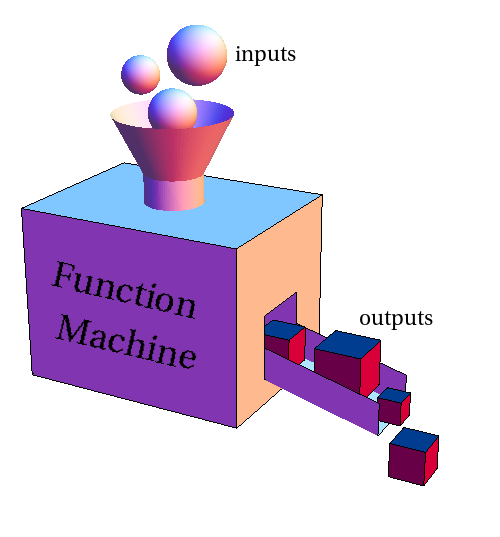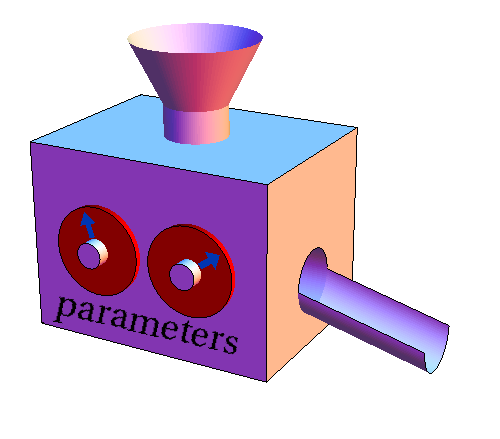# Math Insight

### The exponential function

#### Overview of the exponential function

The exponential function is one of the most important functions in mathematics (though it would have to admit that the linear function ranks even higher in importance). To form an exponential function, we let the independent variable be the exponent. A simple example is the function $$f(x)=2^x.$$As illustrated in the above graph of $f$, the exponential function increases rapidly. Exponential functions are solutions to the simplest types of dynamical systems. For example, an exponential function arises in simple models of bacteria growth

An exponential function can describe growth or decay. The function $$g(x)=\left(\frac{1}{2}\right)^x$$ is an example of exponential decay. It gets rapidly smaller as $x$ increases, as illustrated by its graph.In the exponential growth of $f(x)$, the function doubles every time you add one to its input $x$. In the exponential decay of $g(x)$, the function shrinks in half every time you add one to its input $x$. The presence of this doubling time or half-life is characteristic of exponential functions, indicating how fast they grow or decay.

#### Parameters of the exponential function

As with any function, the action of an exponential function $f(x)$ can be captured by the function machine metaphor that takes inputs $x$ and transforms them into the outputs $f(x)$.The function machine metaphor is useful for introducing parameters into a function. The above exponential functions $f(x)$ and $g(x)$ are two different functions, but they differ only by the change in the base of the exponentiation from 2 to 1/2. We could capture both functions using a single function machine but dials to represent parameters influencing how the machine works.We could represent the base of the exponentiation by a parameter $b$. Then, we could write $f$ as a function with a single parameter (a function machine with a single dial): $$f(x)=b^{x}.$$ When $b=2$, we have our original exponential growth function $f(x)$, and when $b=\frac{1}{2}$, this same $f$ turns into our original exponential decay function $g(x)$. We could think of a function with a parameter as representing a whole family of functions, with one function for each value of the parameter.

We can also change the exponential function by including a constant in the exponent. For example, the function $$h(x)=2^{3x}$$ is also an exponential function. It just grows faster than $f(x)=2^x$ since $h(x)$ doubles every time you add only $1/3$ to its input $x$. We can introduce another parameter $k$ into the definition of the exponential function, giving us two dials to play with. If we call this parameter $k$, we can write our exponential function $f$ as $$f(x)=b^{kx}.$$ You can explore the influence of both parameters $b$ and $k$ in the following applet.

The exponential function. The exponential function $f(x)=b^{kx}$ for base $b >0$ and constant $k$ is plotted in green. You can change the parameters $b$ and $k$ by typing new values in the corresponding boxes. It turns out the parameters $b$ and $k$ can change the function $f$ in the same way, so you really only need to change one of them to see all the different functions $f$. To see how they do the same thing, you can click the “fix function” checkbox, which will fix the function $f(x)$. When that box is checked, if you change the parameters $b$ or $k$, the other parameter will change in a way to leave the function $f(x)$ unchanged. For the function $f(x)=b^{kx}$, the value $f(0)=1$ for all parameters. To change the value of $f(0)$, you can allow scaling of the function by clicking the corresponding checkbox. Then, the function changes to $f(x)=c b^{kx}$ with an additional parameter $c$ that scales (multiplies) the whole function so that $f(0)=c$. You can change the value of $c$ by dragging the red point. You can change range of the $x$ and $y$-axes buttons labeled $x+$, $x-$, $y+$, and $y-$. Since $f(x)$ is always non-negative, only the positive $y$-axis is shown.

It turns out that adding both parameters $b$ and $k$ to our definition of $f$ is really unnecessary. We can still get the full range of functions if we eliminate either $b$ or $k$. You can see this fact through the above applet. For example, you can see that the function $f(x)=3^{2x}$ ($k=2$, $b=3$) is exactly the same as the function $f(x)=9^x$ ($k=1$, $b=9$). In fact, for any change you make to $k$, you can make a compensating change in $b$ to keep the function the same. To see this, check the “fix function” checkbox. Then, if you change either $b$ or $k$, the applet will automatically make a compensatory change in the other parameter to keep the function the same. If you are curious why this is true, you can check out the calculation showing the two parameters are redundant.

Since it is silly to have both parameters $b$ and $k$, we will typically eliminate one of them. The easiest thing to do is eliminate $k$ and go back to the function $$f(x)=b^x.$$ We will use this function a bit at first, changing the base $b$ to make the function grow or decay faster or slower.

However, once you start learning some calculus, you'll see that it is more natural to get rid of the base parameter $b$ and instead use the constant $k$ to make the function grow or decay faster or slower. Except, we can't exactly get rid of the base $b$. If we set $b=1$, we'd have the boring function $f(x)=1$, or, if we set $b=0$, we'd have the even more boring function $f(x)=0$. We need to choose some other value of $b$.

If we didn't have calculus, we'd probably choose $b=2$, writing our exponential function as $f(x)=2^{kx}$. Or, since we like the decimal system so well, maybe we'd choose $b=10$ and write our exponential function of $f(x)=10^{kx}$. According to the above discussion, it shouldn't matter whether we use $b=2$ or $b=10$, as we can get the same functions either way (just with different values of $k$).

But, it turns out that calculus tells us there is a natural choice for the base $b$. Once you learn some calculus, you'll see why the most common base $b$ throughout the sciences is the irrational number $$e= 2.718281828459045 \ldots .$$ Fixing $b=e$, we can write the exponential functions as $$f(x) = e^{kx}.$$ (The applet understands the value of $e$, so you can type $e$ in the box for $b$.) Using $e$ for the base is so common, that $e^x$ (“e to the $x$”) is often referred to simply as the exponential function.

To increase the possibilities for the exponential function, we can add one more parameter $c$ that scales the function: $$f(x)=c b^{kx}.$$ Since $f(0)=cb^{k0} = c$, we can see that the parameter $c$ does something completely different than the parameters $b$ and $k$. We'll often use two parameters for the exponential function: $c$ and one of $b$ or $k$. For example, we might set $k=1$ and use $$f(x)=cb^x$$ or set $b=e$ and use $$f(x)=ce^{kx}.$$ You can add the parameter $c$ to the applet by checking the “scale function” checkbox.